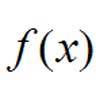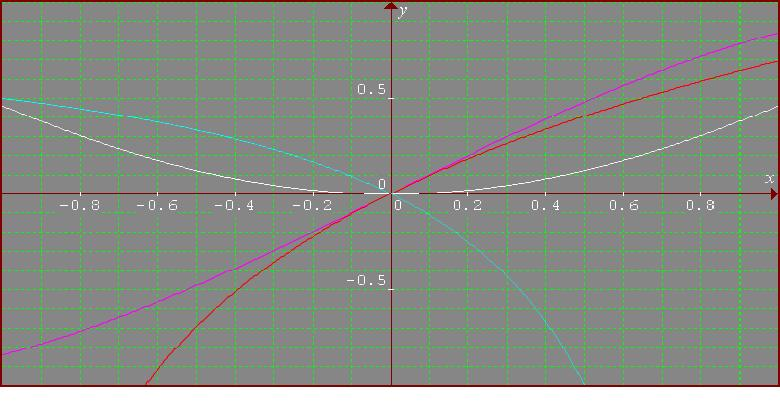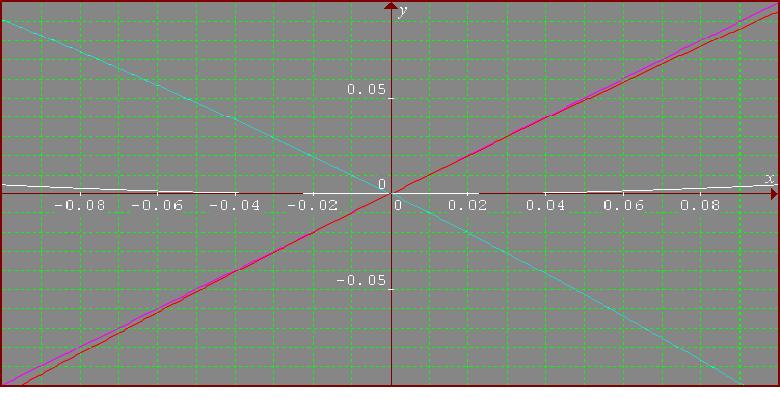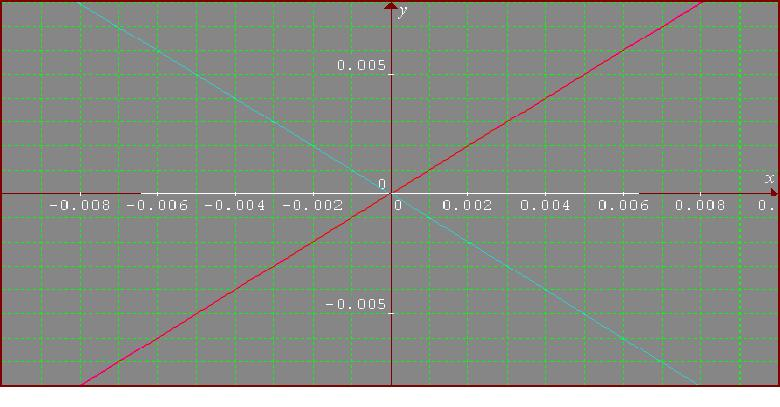#### You may also like### Towards Maclaurin

Build series for the sine and cosine functions by adding one term at a time, alternately making the approximation too big then too small but getting ever closer.### Building Approximations for Sin(x)

Build up the concept of the Taylor series### Taking Trigonometry Series-ly

Look at the advanced way of viewing sin and cos through their power series.

# What Do Functions Do for Tiny X?

##### Age 16 to 18Challenge Level

Congratulations Andrei for another very good solution.

The 4 functions are: $$A(x) = \sin x,\quad B(x) = 1 - \cos x,\quad C(x) = \log (1+x), \quad D(x) = 1- {1\over (1-x)}.$$ I consider the logarithm in base e. First I plotted the 4 functions using Graphmatica. In all figures $A(x)$ is violet, $B(x)$ is white, $C(x)$ is red and $D(x)$ is cyan. For $[-1, 1]$ I obtain:For [-0.1, 0.1]And for [-0.01, 0.01] I have:Each of the 4 functions could be approximated using the Taylor series expansion around 0, and the accuracy of the approximation becomes better for values of $x$ nearer to the origin. I shall use the second order polynomial: $$A(x) \approx x,\quad B(x) \approx 1- (1 - x^2/2) = x^2/2, \quad C(x)\approx x - x^2/2, \quad D(x) \approx - x - x^2.$$